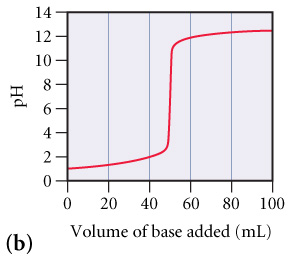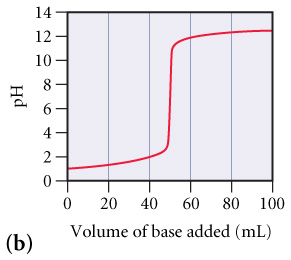# Problem: The graphs below labeled (a) and (b) show the titration curves for two equal-volume samples of monoprotic acids, one weak and one strong. Both titrations were carried out with the same concentration of strong base.What is the approximate pH at the equivalence point of (b) curve?

###### FREE Expert Solution

Determine the approximate pH at the equivalence point of (b) curve:95% (114 ratings)###### Problem Details

The graphs below labeled (a) and (b) show the titration curves for two equal-volume samples of monoprotic acids, one weak and one strong. Both titrations were carried out with the same concentration of strong base.

What is the approximate pH at the equivalence point of (b) curve?Frequently Asked Questions

What scientific concept do you need to know in order to solve this problem?

Our tutors have indicated that to solve this problem you will need to apply the Acid and Base Titration Curves concept. You can view video lessons to learn Acid and Base Titration Curves. Or if you need more Acid and Base Titration Curves practice, you can also practice Acid and Base Titration Curves practice problems.

What professor is this problem relevant for?

Based on our data, we think this problem is relevant for Professor Deshmukh's class at UCSD.